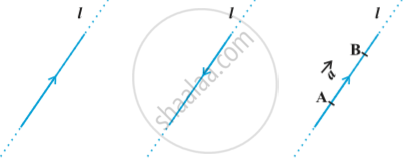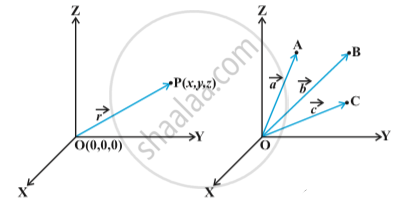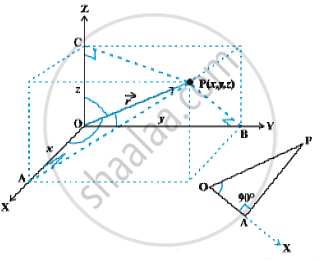Basic Concepts of Vector Algebra

description

• Position Vector
• Direction Cosines and Direction Ratios of a Vector

definition

A quantity that has magnitude as well as direction is called a vector.
A directed line segment is a vector in above  fig., denoted as vec (AB) or simply as vec a , and and read as 'vector vec (AB)' or 'vector veca'.
The point A from where the vector vec (AB) starts is called its initial point, and the point B where it ends is called its terminal point. The distance between initial and terminal points of a vector is called the magnitude (or length) of the vector, denoted as
|vec (AB)|, or |vec a|, or a. The arrow indicates the direction of the vector.

notes

Let ‘l’ be any straight line in plane or three dimensional space. This line can be given two directions by means of arrowheads. A line with one of these directions prescribed is called a directed line in following  first and second fig .Position Vector:
The three dimensional right handed rectangular coordinate system in  following first fig.Consider a point P in space, having coordinates (x, y, z) with respect to the origin O(0, 0, 0). Then, the vector  having O and P as its initial and terminal points, respectively, is called the position vector of the point P with respect to O.
Using distance formula |vec (OP)| = sqrt(x^2 + y^2 + z^2)
In practice, the position vectors of points A, B, C, etc., with respect to the origin O are denoted by vec a , vec b , vec c etc., respectively in above second fig.

Direction Cosines:
The position vector vec (OP) (or vec r) of a point P(x, y, z) as in  following Fig.The angles α, β, γ made by the vector vec r with the positive directions of x, y and z-axes respectively, are called its direction angles.
The cosine values of these angles, i.e., cosα, cosβ and cosγ are called direction cosines of the vector vec r , and usually denoted by l, m and n, respectively.

one may note that the triangle OAP is right angled, and in it, we have cos alpha = x/r( r stands for |vec r|).  Similarly, from the right angled triangles OBP and OCP, we may write  cos beta= y/r and cos gamma = z/r.  Thus, the coordinates of the point P may also be expressed as (lr, mr,nr).  The numbers lr, mr and nr, proportional to the direction cosines are called as direction ratios of vector vec r , and denoted as a, b and c, respectively.

If you would like to contribute notes or other learning material, please submit them using the button below.

Shaalaa.com

Direction cosine and Direction ratio [00:26:35]
S
0%